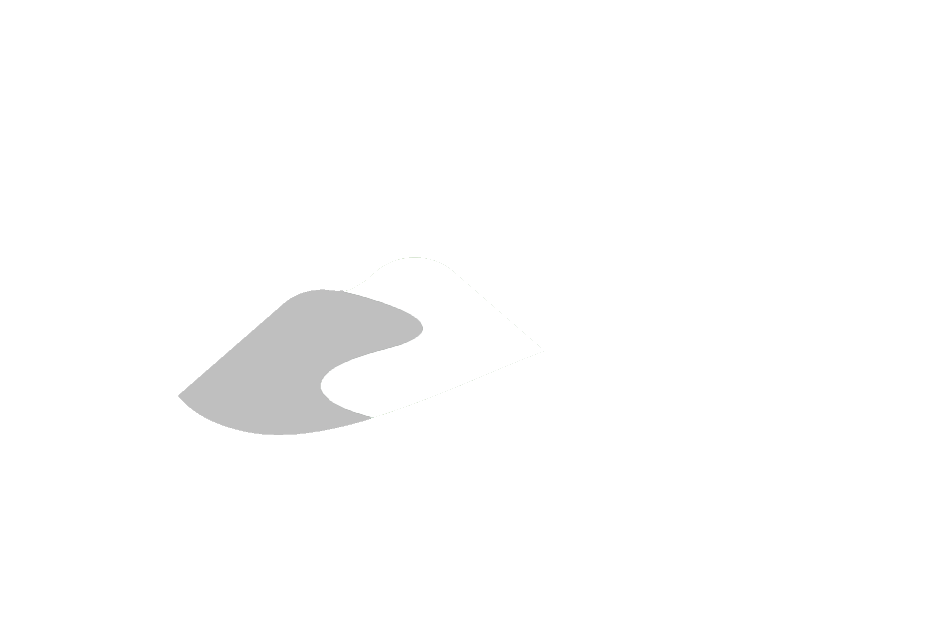# Database Manual

Appendix G.11

## G.11 Correction of D_GEOLOGY_LAYER - Missing Depths or Units

• Tables
• D_GEOLOGY_LAYER
• D_BOREHOLE_CONSTRUCTION
• D_BOREHOLE
• Estimated Recurrence Time: As necessary

When the original units of measure are missing, units are assigned based upon: presence in succeeding layers; presence in D_BOREHOLE_CONSTRUCTION; presence in the original database (e.g. through checking of the original MOE import database); examination of the values themselves (where whole numbers are considered to be ‘fbgs’ and real/decimal numbers considered to be ‘mbgs’; note that this particular point is assumed across multiple table-field checks).

When the original depths are missing, the deepest unit is determined by examination of D_BOREHOLE_CONSTRUCTION and BH_BOTTOM_DEPTH (in D_BOREHOLE) when present. All other layers are taken to be equal intervals down-hole. Comments detailing this process will be present in GEOL_COMMENT (e.g. ‘imposed depth’). In addition, the GEOL_SUBCLASS_CODE will be a tagged with the value of ‘5’ (i.e. ‘Original (Corrected)’). Note that a NULL value in GEOL_SUBCLASS_CODE is assumed to be original/non-modified (i.e. it hasn’t been compared against the original hard copy form).

If no depths are found, the GEOL_SUBCLASS_CODE is given a value of ‘7’ (i.e. ‘Original (Invalid)’).

If the top depth of the last unit is present but the bottom depth is not, it is assumed that the driller stopped at an (hard) interface. This layer is extended with a false bottom of ‘1ft’ (or ‘0.3m’ as appropriate).

If the material codes matches to a surficial material (e.g. topsoil, fill, ?), a depth of ‘1ft’ (or ‘0.3m’ as appropriate) is assumed. The subsequent layer depths (and elevations) should be correct as well.

In some cases, one (or more) layers have a measurement unit that does not correspond to the remainder of the layers. Here, the values modified to match the most likely unit and the depth values are modified appropriately (e.g. whole numbers are assumed, by default, to be ‘fbgs’). The GEOL_SUBCLASS_CODE would be assigned a value of ‘5’.

Where the layers are missing one of top or bottom depths, preceding or succeeding layer depths are used - the difference is split amongst the layers (e.g. a two layer borehole with a depth, of one layer, of ‘30.5’ metres with no other depths indicated; here, a top depth would be applied of ‘15.25’ to the second layer while the first would have a ‘0’ top and a ‘15.25’ bottom).

Where a layer is not contributing any additional information (i.e. no colour or materials) and duplicates depths of other layers, this layer is removed and the remaining layers are marked ‘Simplified’ in the GEOL_COMMENT field.